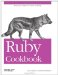# Numbers

Numbers are as fundamental to computing as breath is to human life. Even programs that have nothing to do with math need to count the items in a data structure, display average running times, or use numbers as a source of randomness. Ruby makes it easy to represent numbers, letting you breathe easy and tackle the harder problems of programming.

An issue that comes up when you're programming with numbers is that there are several different implementations of "number," optimized for different purposes: 32bit integers, floating-point numbers, and so on. Ruby tries to hide these details from you, but it's important to know about them because they often manifest as mysteriously incorrect calculations.

 See, for instance, the Discussion section of Recipe 2.11, where it's revealed that Matrix#inverse doesn't work correctly on a matrix full of integers. This is because Matrix#inverse uses division, and integer division works differently from floating-point division.

The first distinction is between small numbers and large ones. If you've used other programming languages, you probably know that you must use different data types to hold small numbers and large numbers (assuming that the language supports large numbers at all). Ruby has different classes for small numbers (Fixnum) and large numbers (Bignum), but you don't usually have to worry about the difference. When you type in a number, Ruby sees how big it is and creates an object of the appropriate class.

```	1000.class # => Fixnum
10000000000.class # => Bignum
(2**30 - 1).class # => Fixnum
(2**30).class # => Bignum
```

When you perform arithmetic, Ruby automatically does any needed conversions. You don't have to worry about the difference between small and large numbers:

 Python also has this feature.

```	small = 1000
big = small ** 5 # => 1000000000000000
big.class # => Bignum
smaller = big / big # => 1
smaller.class # => Fixnum
```

The other major distinction is between whole numbers (integers) and fractional numbers. Like all modern programming languages, Ruby implements the IEEE floating-point standard for representing fractional numbers. If you type a number that includes a decimal point, Ruby creates a Float object instead of a Fixnum or Bignum:

```	0.01.class # => Float
1.0.class # => Float
10000000000.00000000001.class # => Float
```

But floating-point numbers are imprecise (see Recipe 2.2), and they have their own size limits, so Ruby also provides a class that can represent any number with a finite decimal expansion (Recipe 2.3). There's also a class for numbers like two-thirds, which have an infinite decimal expansion (Recipe 2.4), and a class for complex or "irrational" numbers (Recipe 2.12).

Every kind of number in Ruby has its own class (Integer, Bignum, Complex, and so on), which inherits from the Numeric class. All these classes implement the basic arithmetic operations, and in most cases you can mix and match numbers of different types (see Recipe 8.9 for more on how this works). You can reopen these classes to add new capabilities to numbers (see, for instance, Recipe 2.17), but you can't usefully subclass them.

Ruby provides simple ways of generating random numbers (Recipe 2.5) and sequences of numbers (Recipe 2.15). This chapter also covers some simple mathematical algorithms (Recipes 2.7 and 2.11) and statistics (Recipe 2.8).Ruby Cookbook (Cookbooks (OReilly))
ISBN: 0596523696
EAN: 2147483647
Year: N/A
Pages: 399